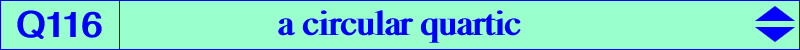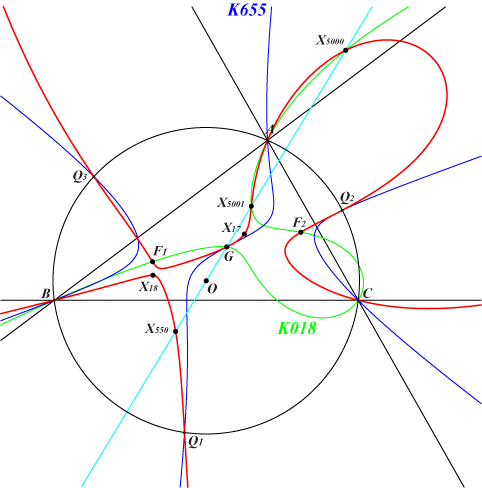too complicated to be written here. Click on the link to download a text file.X(2), X(17), X(18), X(550), X(3413), X(3414), X(5000), X(5001) points of K655 on the circumcircle foci of the Steiner inellipseThe degenerate quartic into K018 and the line at infinity and Q019 generate a pencil of circular quartics which contains the quartics Q115, Q116, Q117, Q118. All these quartics have the same singular focus namely X(187) and their two real asymptotes meet at X on the line X(543)X(625). Here X = 2 a^4-4 a^2 b^2+3 b^4-4 a^2 c^2+3 c^4 : : , with SEARCH = 5.16800530546389.